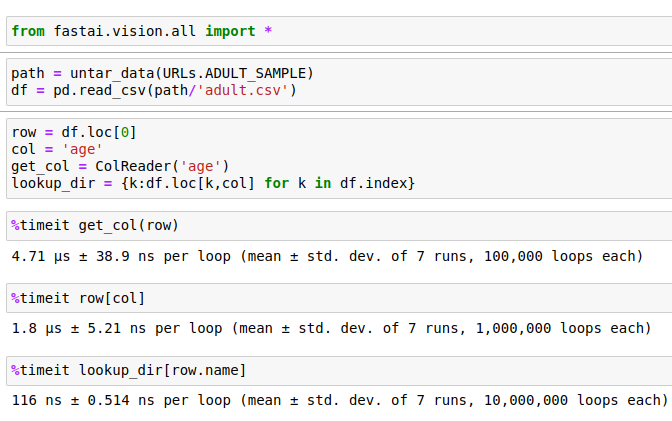# Changing bit depth (bits per pixel) for images / masks

Building a segmentation model with Unet / ResNet. This is my dataloader so far:

``````def src_get_label(fn):
label_name = src_dataset / "GT" / f"{fn.stem}_GT.png"
return label_name

src_datablock = DataBlock(
get_items=get_image_files,
get_y=src_get_label,
splitter=RandomSplitter(seed=42),
item_tfms=Resize(size=input_image_size, method="squish"),
batch_tfms=aug_transforms(),
)

src_dataset / "original", path=src_dataset, bs=src_batch_size
)
``````

The problem is this: the mask files, depending on the dataset, might be in weird formats. Sometimes they are 1 bit per pixel (1 BPP). I can’t always convert them offline to 8 BPP monochrome, which is what the masks in most of my datasets are. In some cases they are 24 BPP full color (RGB).

Same problem, but to a lesser extent, with the actual image files (not masks).

I would like to add processing to the dataloader to convert all images and all masks on the fly to 8 BPP monochrome, which is the format I encounter most often.

Are there any examples showing on the fly image and mask processing where the internal representation of the pixels is changed after the files are loaded but before they are presented to the model?

I’m pretty sure I could handle format detection on the fly, etc, I just need to see how I could approach image file processing in the dataloader.

That’s one of the best parts about fastai: you have an entrypoint to customize stuff at almost every step in the process. If I understand you correctly you should have a closer look at `item_tfms`. Functions added here are applied to every instance after they got loaded as an (PIL.)Image, but before they get batched. Check out this tutorial which should demonstrate something similar to what you want to do.

From your explanation here…

…it sounds like I could also do the mask processing in `get_y` in the DataBlock, since the stuff that passes through there could be an `np.array`.

Case in point, right now I build a Pandas dataframe with columns containing:

• paths to image files
• lists of paths to mask files
• dataset name (for stratification if I train on multiple datasets at once)

My DataBlock right now is:

``````def make_mask(row):
# TODO: merge masks instead of random.choice()
return random.choice(f(row))

src_datablock = DataBlock(
splitter=TrainTestSplitter(stratify=src_df["dataset"].to_list(), random_state=42),
item_tfms=Resize(size=input_image_size, method="squish"),
batch_tfms=aug_transforms(),
)
``````

If I understand your explanations in both threads correctly, then I could have a custom function in `get_y` which:

• receives a list of paths to mask files (usually the list has just one item, occasionally has multiples)
• grabs each file, converts it to `np.array`
• if the format is not `np.uint8` then converts it (the problem in this thread)
• if there are multiple files, merge the masks (the problem in the other thread)
• return the result to the DataBlock

I will try something along these lines.

Or I could just modify `make_mask()` since it is called on the list of masks anyway, and it appears (???) to be equivalent with calling `get_x` and `get_y` separately.

I have this and it appears to work:

``````def make_mask2(row):
all_images = [np.asarray(PILMask.create(x)) for x in f(row)]
image_stack = np.stack(all_images)
image_union = np.amax(image_stack, axis=0)

src_datablock = DataBlock(
splitter=TrainTestSplitter(stratify=src_df["dataset"].to_list(), random_state=42),
item_tfms=Resize(size=input_image_size, method="squish"),
batch_tfms=aug_transforms(),
)
``````

It collapses multiple masks into a single mask. It ensures a consistent 8 bits per pixel format for the masks. The part that ensures 8 BPP appears to be `PILMask.create()` (the default there seems to be 8 BPP).

The function is likely not optimal in terms of speed.

Hey, that seems sensible to meRegarding your concerne about speed, I don’t know if there is much potential, since you have to load every mask, merge them, make a “fastai/torch-object”…
Two things that you could check if it does make a difference is:
Get the list of masks without `ColRead`. I made this very quick comparison:maybe thats worth a try.
The other idea would be to use parallelization to load the mask, but I don’t know enough about this to know if this actually makes sense(or point you in any further direction).

1 Like